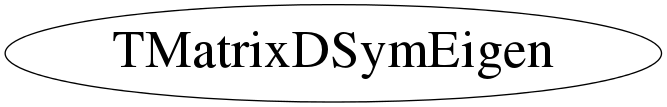# class TMatrixDSymEigen

```
TMatrixDSymEigen

Eigenvalues and eigenvectors of a real symmetric matrix.

If A is symmetric, then A = V*D*V' where the eigenvalue matrix D is
diagonal and the eigenvector matrix V is orthogonal. That is, the
diagonal values of D are the eigenvalues, and V*V' = I, where I is
the identity matrix.  The columns of V represent the eigenvectors in
the sense that A*V = V*D.

```

## Function Members (Methods)

public:
 virtual ~TMatrixDSymEigen() static TClass* Class() const TVectorD& GetEigenValues() const const TMatrixD& GetEigenVectors() const virtual TClass* IsA() const TMatrixDSymEigen& operator=(const TMatrixDSymEigen& source) virtual void ShowMembers(TMemberInspector& insp) const virtual void Streamer(TBuffer&) void StreamerNVirtual(TBuffer& ClassDef_StreamerNVirtual_b) TMatrixDSymEigen() TMatrixDSymEigen(const TMatrixDSym& a) TMatrixDSymEigen(const TMatrixDSymEigen& another)
protected:
 static void MakeEigenVectors(TMatrixD& v, TVectorD& d, TVectorD& e) static void MakeTridiagonal(TMatrixD& v, TVectorD& d, TVectorD& e)

## Data Members

public:
 static TMatrixDSymEigen::(anonymous) kWorkMax
protected:
 TVectorD fEigenValues Eigen-values TMatrixD fEigenVectors Eigen-vectors of matrix

## Class Charts## Function documentation

TMatrixDSymEigen(const TMatrixDSym& a)
``` Constructor for eigen-problem of symmetric matrix A .
```
TMatrixDSymEigen(const TMatrixDSymEigen& another)
``` Copy constructor
```
void MakeTridiagonal(TMatrixD& v, TVectorD& d, TVectorD& e)
``` This is derived from the Algol procedures tred2 by Bowdler, Martin, Reinsch, and
Wilkinson, Handbook for Auto. Comp., Vol.ii-Linear Algebra, and the corresponding
Fortran subroutine in EISPACK.
```
void MakeEigenVectors(TMatrixD& v, TVectorD& d, TVectorD& e)
``` Symmetric tridiagonal QL algorithm.
This is derived from the Algol procedures tql2, by Bowdler, Martin, Reinsch, and
Wilkinson, Handbook for Auto. Comp., Vol.ii-Linear Algebra, and the corresponding
Fortran subroutine in EISPACK.
```
TMatrixDSymEigen & operator=(const TMatrixDSymEigen& source)
``` Assignment operator
```

`{}`
virtual ~TMatrixDSymEigen()
`{}`
const TMatrixD & GetEigenVectors() const
``` If matrix A has shape (rowLwb,rowUpb,rowLwb,rowUpb), then each eigen-vector
must have an index running between (rowLwb,rowUpb) .
For convenience, the column index of the eigen-vector matrix
also runs from rowLwb to rowUpb so that the returned matrix
has also index/shape (rowLwb,rowUpb,rowLwb,rowUpb) .
The same is true for the eigen-value vector .
```
`{ return fEigenVectors; }`
const TVectorD & GetEigenValues() const
`{ return fEigenValues; }`